Next: Derivation of Darwin-Radau equation Up: Darwin-Radau equation Previous: Derivation and properties of

Let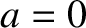(D.33)

It follows that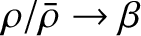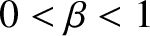(D.34) and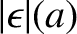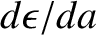(D.35)

Equation (D.28) transforms into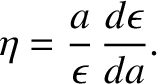(D.36)

Making use of Equation (D.27), this reduces to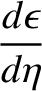(D.37)

However, it is easily demonstrated that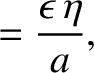(D.38)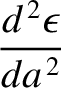(D.39)

where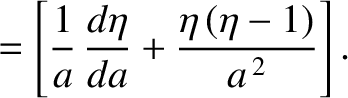(D.40)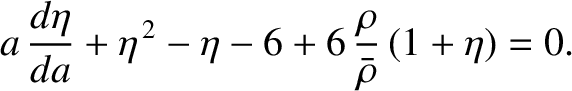Figure D.1 shows the function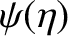. It should be noted that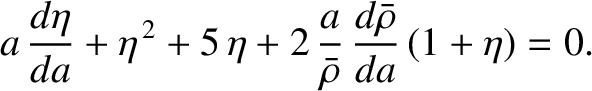is necessarily positive, because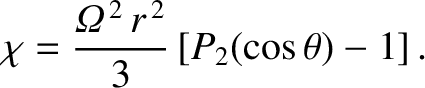is a monotonically increasing function. Moreover,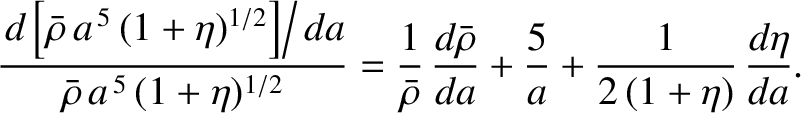on the surface of the Earth, and the largest value of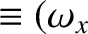for any planet in the solar system is about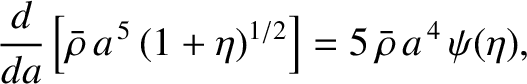(Cook 1980). It is thus clear, from the figure, that it is an excellent approximation to take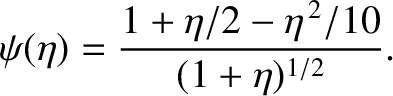for such bodies. This leads to the so-called reduced Radau equation,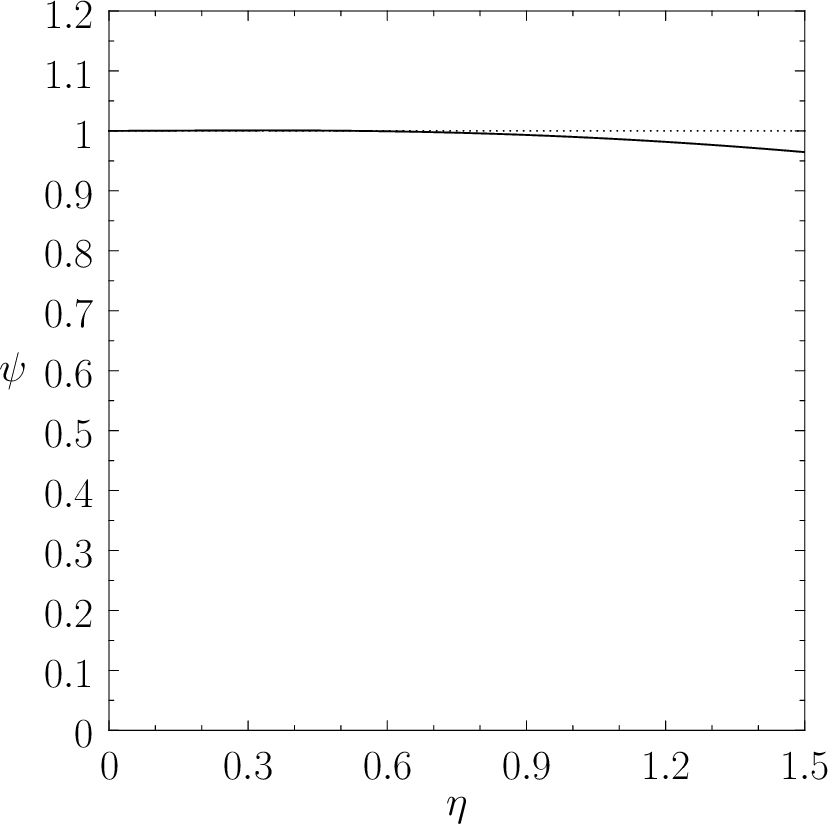(D.41)

which can immediately be integrated to give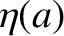(D.42)Next: Derivation of Darwin-Radau equation Up: Darwin-Radau equation Previous: Derivation and properties of
Richard Fitzpatrick 2016-03-31